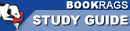Study Guide

# The Logic of Scientific Discovery - Part II: Chapter 8, Probability Summary & Analysis

This Study Guide consists of approximately 34 pages of chapter summaries, quotes, character analysis, themes, and more - everything you need to sharpen your knowledge of The Logic of Scientific Discovery.
 This section contains 1,808 words (approx. 5 pages at 400 words per page) View a FREE sample## Part II: Chapter 8, Probability Summary and Analysis

Discussions of probability can be applied to many subjects. Popper limits his discussion in this chapter to the probability of events and associated problems,as shown in games of chance and with the probabilistic laws of physics. He begins with a basic problem with probability: although probability statements play a vitally important role in empirical science, they turn out in principle to be outside the concept of falsification. His goal in this chapter is to test his theory against this problem. He does this by providing a new foundation for the calculus of probability as a new frequency theory, and by exploring the relationships between probability and experience.

First he distinguishes between two types of probability, numerical and non-numerical probability. Numerical probability involves considering the number of favorable cases divided by the number of possible...

(read more from the Part II: Chapter 8, Probability Summary)

 This section contains 1,808 words (approx. 5 pages at 400 words per page) View a FREE sampleMore summaries and resources for teaching or studying The Logic of Scientific Discovery.